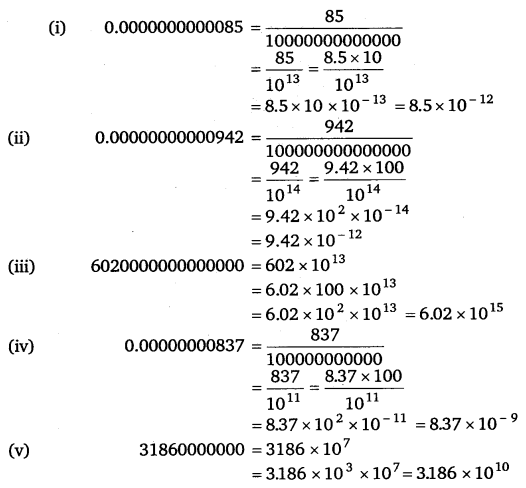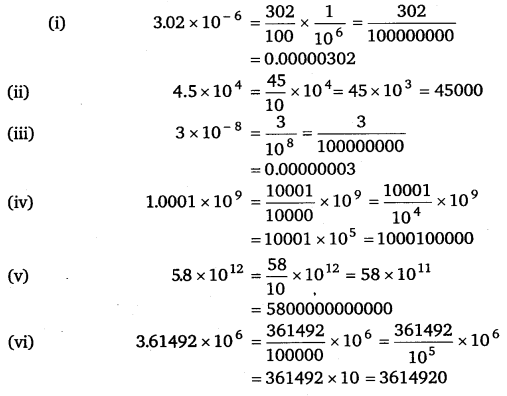# Class 8 Maths NCERT Solutions for Chapter – 12 Exponents and Powers Ex – 12.2

## Exponents and Powers

Question 1.
Express the following numbers in standard form
(i)
0.0000000000085
(ii) 0.00000000000942
(iii) 6020000000000000
(iv) 0.00000000837
(v) 31860000000

Solution:Question 2.
Express the following
(i)
3.02 x 10-6
(ii) 45 x 104
(iii) 3 x 10-8
(iv) 1.0001 x 109
(v) 5.8 x 1012
(vi) 3.61492 x 106

Solution:Question 3.
Express the number appearing in the following statements in standard form :
(i)
1 micron is equal to$\frac { 1 }{ 1000000 }$ m.
(ii) Charge of an electron is 0.00000000000000000016 coulomb.
(iii) Size of a bacteria is 0.0000005 m.
(iv) Size of a plant cell is 0.00001275 m.
(v) Thickness of a thick paper is 0.07 mm.

Solution:Question 4.
In a stack there are 5 books each of thickness 20 mm and 5 paper sheets each of thickness 0.016 mm. What is the total thickness of the stack?

Solution:
Thickness of one book =20 mm
∴ Thickness of 5 books = 5 x 20 mm = 100 mm
Thickness of 1 sheet of paper =0.016 mm
Thickness of 5 sheets of paper = 5 x 0.016 mm = 0.08 mm
Total thickness = 100 mm + 0.08 mm
= 100.08 mm = 1.0008 x 102 mm